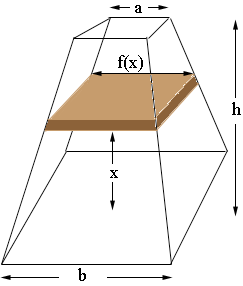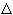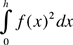SEARCH HOMEMath Central Quandaries & QueriesSubject: Integration Name: Sam Who are you: Student Find the volume of a frustum of a pyramid with square base of side b, square top of side a, and height h. Thanks for the help.Hi Sam,

Imagine that you move up from the base x units and cut a horizontal slice through the pyramid as in the diagram.Suppose that the slice is of thicknessx. The top of the slice is a square and let f(x) be the length of a side of the square. Hence the volume of the slice is approximately

f(x)2x square units.

From integration theory you can now conclude that the volume of the frustum isAll that remains is to find f(x). That you can accomplish using similar triangles.

PennyMath Central is supported by the University of Regina and The Pacific Institute for the Mathematical Sciences.